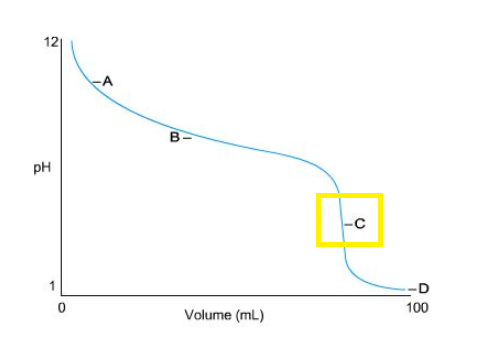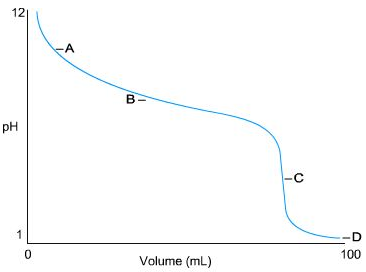# Problem: Identify the equivalence point on the titration curve shown here. a) A is the equivalence point b) B is the equivalence paint c) C is the equivalence point d) D is the equivalence pointDefine the end point of a titration. a) It is the point at which the pH no longer changes. b) It is when a change that indicates equivalence is observed in the analyte solution. c) It is a synonym for equivalence point.

###### FREE Expert Solution

Equivalence point → point in a titration graph where pH changes abruptly

Notice that pH changes at point C:82% (448 ratings)###### Problem DetailsIdentify the equivalence point on the titration curve shown here.

a) A is the equivalence point

b) B is the equivalence paint

c) C is the equivalence point

d) D is the equivalence point

Define the end point of a titration.

a) It is the point at which the pH no longer changes.

b) It is when a change that indicates equivalence is observed in the analyte solution.

c) It is a synonym for equivalence point.